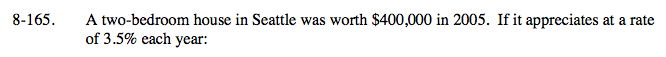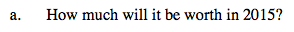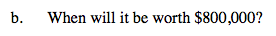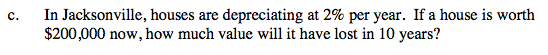Home > A2C > Chapter 8 > Lesson 8.2.4 > Problem8-165

8-165.
1. A two-bedroom house in Seattle was worth $400,000 in 2005. If it appreciates at a rate of 3.5% each year: Homework Help ✎ 1. How much will it be worth in 2015? 2. When will it be worth$800,000?

3. In Jacksonville, houses are depreciating at 2% per year. If a house is worth $200,000 now, how much value will it have lost in 10 years?Write an equation based on the general exponential equation: y = abx About$564,240In the equation you found in part (a), let y = 800,000.

In 2025.The multiplier is NOT 0.02. What percent of the value of a home remains each year if it is depreciating at 2%?